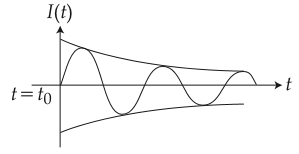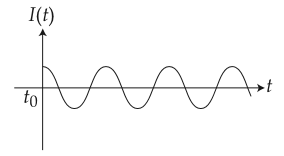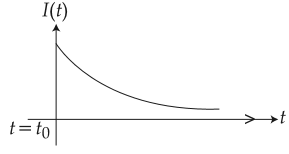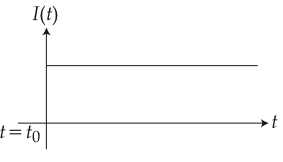#### A series LR circuit is connected to a voltage source with V(t) = V0  sint.  After very large time, current  I(t)  behaves asOption 1)Option 2)Option 3)Option 4)As we learnt in

Equation of V -- whereinAngular frequency= Frequency

T =  time period=Option 1)This option is incorrect.

Option 2)This option is correct.

Option 3)This option is incorrect.

Option 4)This option is incorrect.

#### Plabita